# NCERT Solutions For Class 10 Maths Chapter 6 Exercise 6.5

## Chapter 6 Ex.6.5 Question 1

Sides of triangles are given below. Determine which of them are right triangles.

In case of a right triangle, write the length of its hypotenuse.

(i)  $$7 \;\rm{cm}, 24 \;\rm{cm}, 25\; \rm{cm}$$

(ii)  $$3\; \rm{cm}, 8 \;\rm{cm}, 6 \;\rm{cm}$$

(iii)  $$50\; \rm{cm}, 80 \;\rm{cm}, 100 \;\rm{cm}$$

(iv)  $$13\; \rm{cm}, 12 \;\rm{cm}, 5 \;\rm{cm}$$

### Solution

Reasoning:

As we know,in a triangle, the square of one side is equal to the sum of the squares of the other two sides,then the angle opposite the first side is a right angle.

Steps:

(i) $${{(25)}^{2}} =625$$

\begin{align} {{7}^{2}}+{{24}^{2}}&=49+576 \\&=625 \\ \therefore \,\,\,\,{{(25)}^{2}}&={{7}^{2}}+{{24}^{2}} \\ \end{align}

Length of hypotenuse $$=$$ $$25\;\rm{cm}$$

(ii) $${{8}^{2}} =64$$

\begin{align} {{3}^{2}}+{{6}^{2}}&=9+36 \\ &=45 \\ {{8}^{2}}&\ne {{3}^{2}}+{{6}^{2}} \\ \end{align}

(iii) $${{(100)}^{2}}=1000$$

\begin{align} {{50}^{2}}+{{80}^{2}}&=2500+6400 \\ &=8900 \\ {{(100)}^{2}}&\ne {{50}^{2}}+{{80}^{2}} \\ \end{align}

(iv) $${{\left( 13 \right)}^{2}}=169$$

\begin{align} {{12}^{2}}+{{5}^{2}}&=144+25 \\ &=169 \\ \end{align}

$$\therefore{{(13)}^{2}}={{12}^{2}}+{{5}^{2}}$$

Length of hypotenuse $$=$$ $$13\;\rm{cm}$$

$$\Rightarrow$$ (i) and (iv) are right triangle.

## Chapter 6 Ex.6.5 Question 2

$${PQR}$$ is a triangle right angled at $$P$$ and $$M$$ is a point on $$QR$$ such that $$PM \bot QR$$. Show that $${PM}^2=QM.MR$$

Diagram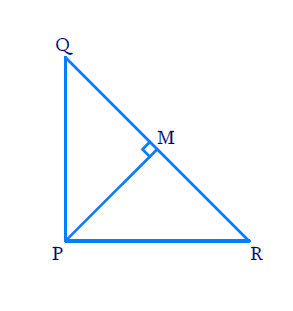### Solution

Reasoning:

As we know if a perpendicular is drawn from the vertex of the right angle of a right triangle to the hypotenuse then triangles on both sides of the perpendicular are similar to the whole triangle and to each other.

Steps:

In $$\Delta PQR\,;\,\angle QPR={{90}^{0}}$$ and $$PM\bot QR$$

In $$\,\Delta PQR\,$$ and $$\,\Delta MQP\,$$

\begin{align} & \angle Q P R=\angle Q M P=\,90^{\circ} \\ & \angle P Q R=\angle M Q P \\ & \text{(Common Angles)} \\\\ \Rightarrow \quad & \Delta P Q R \sim \Delta M Q P \\ & \text{(AA Criterion)} \qquad\dots (1) \end{align}

In $$\Delta PQR$$ and $$\Delta MPR$$

\begin{align} & \angle QMP=\angle PMR=90^\circ \\ & \angle PRQ=\angle RPM \\ & \text{(Common Angles)} \\\\ \Rightarrow\quad &\Delta \,PQR \sim{\ }\Delta \,MPR \\ & \text{(AA Criterion)} \qquad\dots (2) \end{align}

From $$(1)$$ and $$(2)$$

\begin{align}&\Delta MQP\sim{\ }\Delta MPR \\&\frac{P M}{M R}=\frac{Q M}{PM}\\&\begin{bmatrix}\text{Comparing sides }\\\text{opposite to equal angles}\end{bmatrix}\\\\\Rightarrow\quad &P M^{2}=Q M \cdot M R\end{align}

## Chapter 6 Ex.6.5 Question 3

In Figure, $${ABD}$$ is a triangle right angled at $$A$$ and $${AC⊥BD}$$. Show that

(i) $$A{{B}^{2}}=BC.BD$$

(ii) $$A{{C}^{2}}=BC.DC$$

(iii) $$A{{D}^{2}}=BD.CD$$

Diagram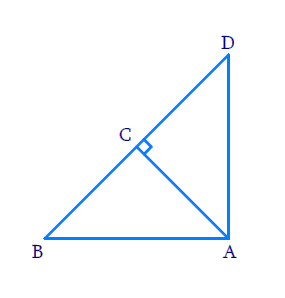### Solution

Reasoning:

As we know if a perpendicular is drawn from the vertex of the right angle of a right triangle to the hypotenuse then triangles on both sides of the perpendicular are similar to the whole triangle and to each other.

Steps:

i) In $$\Delta BAD\;\text{and}\;\Delta BCA$$

\begin{align} & \Rightarrow \Delta BAD \sim \Delta BCA \\ & \\ & \Rightarrow \frac{AB}{BC}=\frac{BD}{AB} \\ & \left[ \begin{array} & \text{Corresponding sides } \\ \text{of similar triangle} \\ \end{array} \right] \\ & \\ & \Rightarrow A{{B}^{2}}=BC\cdot BD \\ \end{align}

ii) In $$\Delta BCA\,\,\text{and}\,\Delta ACD$$

\begin{align} & \Rightarrow \Delta BCA \sim\Delta ACD \\ & \\ & \Rightarrow \frac{AC}{CD}=\frac{BC}{AC}\qquad \\ & \left[ \begin{array} & \text{Corresponding sides } \\ \text{of similar triangle} \\ \end{array} \right] \\ & \\ & \Rightarrow A{{C}^{2}}=BC\cdot DC \\ \end{align}

iii) In $$\Delta BAD$$ and $$\Delta ACD$$

\begin{align} & \Rightarrow \Delta BAD \sim \Delta ACD \\ & \\ & \Rightarrow \frac{AD}{CD}=\frac{BD}{AD}\qquad \\ & \left[ \begin{array} & \text{Corresponding sides} \\ \text{ of similar triangle} \\ \end{array} \right] \\ & \\ & A{{D}^{2}}=BD\cdot CD \\ \end{align}

## Chapter 6 Ex.6.5 Question 4

$$ABC$$ is an isosceles triangle right angled at $$C.$$ Prove that $${AB}^{2}=2{AC}^{2}$$.

Diagram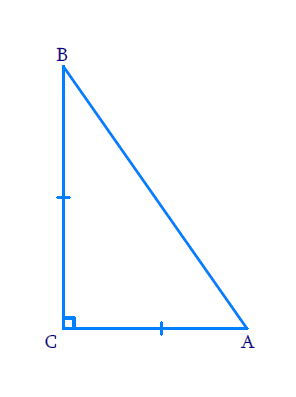### Solution

Reasoning:

As we are aware, in a right triangle, the square of the hypotenuse is equal to the sum of the squares of the other two sides.

Steps:

In $$\Delta ABC,\;\angle ACB=\,90^{\circ}$$ and $$AC=BC$$

But

\begin{align} A{{B}^{2}}&=A{{C}^{2}}+B{{C}^{2}} \\ A{{B}^{2}}& =A{{C}^{2}}+A{{C}^{2}}\;\;[\because AC=BC] \\ A{{B}^{2}}&=2A{{C}^{2}} \end{align}

## Chapter 6 Ex.6.5 Question 5

$$ABC$$ is an isosceles triangle with $${AC = BC}$$. If $$A B^{2}=2 A C^{2}$$ prove that $$ABC$$ is a right triangle.

Diagram### Solution

Reasoning:

As we know ,In a triangle, if square of one side is equal to the sum of the squares of the other two sides, then the angle opposite the first side is a right angle.

Steps:

In $$\Delta A B C$$

$AC = BC$

And

\begin{align}A B^{2} &=2 A C^{2} \\ &=A C^{2}+A C^{2} \\ A B^{2} &=A C^{2}+B C^{2} \quad[\because A C=B C] \end{align}

$$\Rightarrow \angle A C B=90^\circ$$

\begin{align}\Rightarrow \Delta A B C \end{align} is a right triangle

## Chapter 6 Ex.6.5 Question 6

$$ABC$$ is an equilateral triangle of side $$2$$$$a.$$ Find each of its altitudes.

Diagram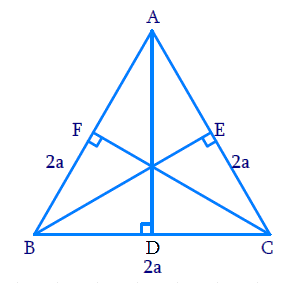### Solution

Reasoning:

We know that in an equilateral triangle perpendicular drawn from vertex to the opposite side, bisects the side.

As we know that, in a right triangle, the square of the hypotenuse is equal to the sum of the squares of the other two sides.

Steps:

In $$\Delta ABC$$

$$A B=B C=C A=2 a$$

$$A D \perp B C$$

\begin{align}\Rightarrow\; B D&=C D=\frac{{1}}{2}BC={a}\end{align}

In $$\Delta ADB$$ ,

\begin{align} A B^{2} &=A D^{2}+B D^{2} \\ A D^{2} &=A B^{2}-B D^{2} \\ &=(2 a)^{2}-a^{2} \\ &=4 a^{2}-a^{2} \\ &=3 a^{2} \\AD &=\sqrt{3} a\\ \Rightarrow A D &=\sqrt{3} a \text { Units } \end{align}

Similarly, we can prove that

$BE=CF=\sqrt{3} a \text { Units }$

## Chapter 6 Ex.6.5 Question 7

Prove that the sum of the squares of the sides of a rhombus is equal to the sum of the squares of its diagonals.

Diagram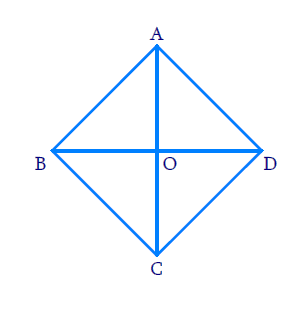### Solution

Reasoning:

As we know, In a rhombus , diagonals bisect each other perpendicularly.

Steps:

In rhombus $${ABCD}$$

$$AC\bot BD$$ and $$OA = OC ;OB = OD$$

In $$\Delta AOB$$

\begin{align}\angle A O B=90^{\circ} \end{align}

$$\Rightarrow A B^{2}=O A^{2}+O B^{2} \;\;\dots (1)$$

Similarly, we can prove

\begin{align} B{{C}^{2}}=O{{B}^{2}}+O{{C}^{2}}\;\;\dots \left( 2 \right) \\ C{{D}^{2}}=O{{C}^{2}}+O{{D}^{2}}\;\;\dots \left( 3 \right) \\ A{{D}^{2}}=O{{D}^{2}}+O{{A}^{2}}\;\;\dots \left( 4 \right) \\ \end{align}

Adding $$(1), (2), (3)$$ and $$(4)$$

\begin{align} &\;\;\;\; A{B^2} + B{C^2} + C{D^2} + A{D^2} \\& = \!\! \begin{bmatrix} \! OA^2 \! + \! OB^2 \! +\! OB^2 \!+ \!OC^2 +\! \\ \! OC^2 \! + \! OD^2 \! + \! OD^2 \! + \! OA^2 \end{bmatrix} \\ & \!= \!\! 2OA^2 \!\! + \!2O{B^2} \!+\! 2OC^2 \!+\! 2OD^2 \\ &\!= \!\!2 [O{A^2} \!+\! O{B^2} \!+\! O{C^2} \!+ \! O{D^2}] \\ &\!= \!\!2 \left[ \! \!\left( \frac{AC}{2} \right)^2 \!\! +\! \left( \frac{BD}{2} \right)^2 \! + \! \left( \frac{AC}{2} \right)^2 +\! \left( \frac{BD}{2} \right)^2 \right] \\\\ & \qquad OA = OC = \frac{{AC}}{2} \\\\ &\qquad \qquad \quad \text{and} \\\\& \qquad OB = OD = \frac{BD}{2} \\\\ &= 2 \left[ \frac{ AC^2 + BD^2 + AC^2 + BD^2 }{4} \right] \\ &= 2 \left[ \frac{2AC^2 + 2BD^2}{4}\right] \\ &= 4\left[ {\frac{{A{C^2} + B{D^2}}}{4}} \right]\\ &= A{C^2} + B{D^2} \end{align}

\begin{align} \therefore AB^2 \!\!+\!\! BC^2 \!\! + \!\! CD^2 \!\!+ \!\! AD^2 & \!\!=\!\! AC^2 \!\!+ \!\! BD^2 \end{align}

## Chapter 6 Ex.6.5 Question 8

In Figure, $$O$$ is a point in the interior of  $$\Delta ABC,$$ $$OD \bot BC, OE \bot AC$$ and $$OF \bot AB$$. Show that

(i)

\begin{align} & \begin{bmatrix} O{A}^{2}\!\! +\!\! O{B}^{2}\!\!+ \!\! O{C}^{2} \!\! -\! \! O{D}^{2} \!\! - O{E}^{2}\!- \! O{F}^{2}\end{bmatrix} \\ & = AF^{2} +B{D}^{2} +C{E}^{2} \end{align}

(ii)

\begin{align} & AF{}^{2}+B{{D}^{2}} +C{{E}^{2}} \\& = AE{}^{2} +C{{D}^{2}} +B{{F}^{2}} \end{align}

Diagram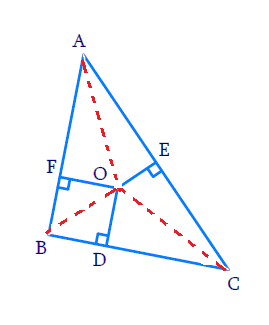### Solution

Reasoning:

In a right triangle, the square of the hypotenuse is equal to the sum of the squares of the other two sides.

Steps:

(i) In $$\,\Delta ABC$$

$$OD\bot BC\,,\,OE\bot AC$$ and $$OF\bot AB$$

$$OA , OB\;\;$$   and $$OC\;\;$$   joined

In $$\,\Delta OAF$$

\begin{align}O{{A}^{2}} & =AF{}^{2}+O{{F}^{2}} \cdots (1)\\ & \quad [\because \angle OFA={{90}^{0}}] \end{align}

Similarly, In $$\,\Delta OBD$$

\begin{align} O{{B}^{2}}& =BD{}^{2}+O{{D}^{2}} \cdots (2) \\ & \quad [\because \angle ODA={{90}^{0}}] \end{align}

In $$\,\Delta OCE$$

\begin{align}O{{C}^{2}}&=C{{E}^{2}}+O{{E}^{2}} \cdots (3) \\ & \quad \left[ \because \, \angle OEC={{90}^{\circ }} \right] \end{align}

Adding $$(1), (2)$$ and $$(3)$$

\begin{align} & O{{A}^{2}} +O{{B}^{2}} +O{{C}^{2}} \\ &= \begin{bmatrix} A{{F}^{2}} +O{{F}^{2}}+B{{D}^{2}} +O{{D}^{2}} \\ +C{{E}^{2}} +O{{E}^{2}} \end{bmatrix} \\ & \;\;\;\;\; \begin{bmatrix} O{{A}^{2}} +O{{B}^{2}} +O{{C}^{2}} -O{{D}^{2}} \\ -O{{E}^{2}} -O{{F}^{2}} \end{bmatrix} \\ &= A{{F}^{2}} +B{{D}^{2}} +C{{E}^{2}} \qquad \cdots(4) \\ \end{align}

(ii) From $$(4)$$

\begin{align} & \begin{bmatrix} (O{{A}^{2}}- \! O{{E}^{2}}) \!+ \! (O{{B}^{2}} - \!O{{F}^{2}}) \! \\+ \! (O{{C}^{2}}-O{{D}^{2}}) \end{bmatrix} \\ & = AF{}^{2}+B{{D}^{2}}+C{{E}^{2}}\\ &\text{(Rearranging the left side terms)} \end{align}

\begin{align} & AE{}^{2}+B{{F}^{2}} +C{{D}^{2}} \\ & = AF{}^{2}+B{{D}^{2}} +C{{E}^{2}} \end{align}

[$$\because\Delta OAE,\Delta OBD$$  and  $$\Delta OCE$$ are right triangles]

## Chapter 6 Ex.6.5 Question 9

A ladder $$10$$$$\rm{}m$$ long reaches a window $$8$$$$\rm{}m$$ above the ground. Find the distance of the foot of the ladder from base of the wall.

Diagram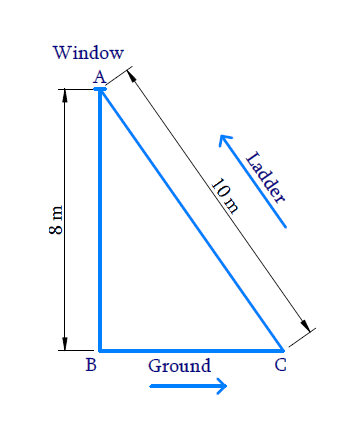### Solution

Reasoning:

In a right triangle, the square of the hypotenuse is equal to the sum of the squares of the other two sides.

Steps:

$$AB$$ is height of the windows from the ground $$\rm=8\,m$$

$$AC$$ is the length of the ladder $$\rm=10\,m$$

$$BC$$ is the foot of the ladder from the base of ground $$= ?$$

Since $$\Delta ABC$$ is right angled triangle $$(\angle ABC={{90}^{0}})$$

\begin{align} \Rightarrow B C^{2} &=A C^{2}-A B^{2} \\ [ \text { Pythagoras } &\text{ theorem } ] \\\\ B C^{2}&=10^{2}-8^{2} \\ B C^{2}&=100-64 \\ B C^{2} &=36 \\ B C &=6 \rm{}m \end{align}

The distance of the foot of the ladder from the base of the wall $$\rm=6\,m$$

## Chapter 6 Ex.6.5 Question 10

A guy wire attached to a vertical pole of height $$18$$$$\rm{}m$$ is $$24$$ $$\rm{}m$$ long and has a stake attached to the other end. How far from the base of the pole should the stake be driven so that the wire will be taut?

Diagram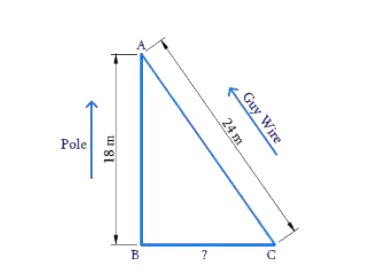### Solution

Reasoning:

In a right triangle, the square of the hypotenuse is equal to the sum of the squares of the other two sides.

Steps:

$$AB$$ is the length of the pole $$\rm = 18\,m$$

$$AC$$ is the length of the guy wire $$\rm = 24\,m$$

$$BC$$ is the distance of the stake from the pole $$= ?$$

In $$\Delta ABC\,\,\,\,\,\angle ABC={{90}^{0}}$$

\begin{align} B C^{2} &=A C^{2}-A B^{2} \\ & [ \text { Pythagoras } \text{ theorem } ] \\\\ B C^{2}&=24^{2}-18^{2} \\ B C^{2}&=576-324 \\ B C^{2}&=252 \\ B C &=2 \times 3 \sqrt{7} \\ B C &=6 \sqrt{7} \end{align}

The distance of the stake from the pole $$=6\sqrt{7}\rm{}m$$

## Chapter 6 Ex.6.5 Question 11

An aeroplane leaves an airport and flies due north at a speed of $$1000\; \rm{}km$$ per hour. At the same time, another aeroplane leaves the same airport and flies due west at a speed of $$1200\; \rm{}km$$ per hour. How far apart will be the two planes after $$\,1\frac{1}{2}$$ hours?

Diagram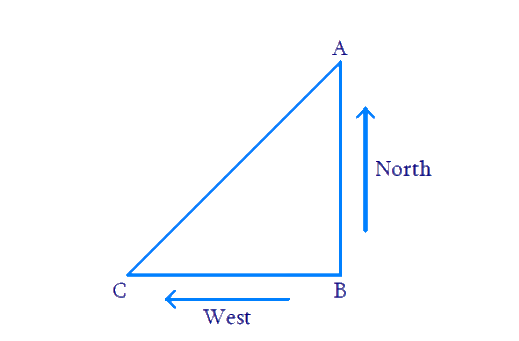### Solution

Reasoning:

We have to find the distance travelled by aeroplanes, we need to use

$\text{Distance}= \text{Speed} \times \text{Time}$

In a right triangle, the square of the hypotenuse is equal to the sum of the squares of the other two sides.

Steps:

$$AB$$ is the distance travelled by aeroplanes travelling towards north

\begin{align} A B &=1000\, \mathrm{km} / \mathrm{hr} \times 1 \frac{1}{2} \mathrm{hr} \\ &=1000 \times \frac{3}{2} \mathrm{km} \\ {AB} &=1500\, \mathrm{km} \end{align}

$$BC$$ is the distance travelled by another aeroplane travelling towards south

\begin{align} BC&=1200\,\,\text{km/hr}\times 1\frac{1}{2}\,\,\text{hr} \\ \,\,\,\,\,\,\,\,\,\,&=1200\times \frac{3}{2}\text{hr} \\ BC&=1800\,\,\text{km} \\ \end{align}

Now, In $$\Delta ABC\,\,,\,\,\angle ABC={{90}^{0}}$$

\begin{align} AC{}^{2}&=A{{B}^{2}}+B{{C}^{2}}\\ & [\text{Pythagoras }\text{theorem}] \\\\ & ={{(1500)}^{2}}+{{(1800)}^{2}} \\ & =2250000+3240000 \\ A{{C}^{2}}&=5490000 \\ AC&=\sqrt{549000} \\ \,\,\,\,\,\,\,\,\,\,\,&=300\sqrt{61}\,\,\text{km} \end{align}

The distance between two planes after  $$1\frac{1}{2}\text{hr}=300\sqrt{61}\,\,\text{km}$$

## Chapter 6 Ex.6.5 Question 12

Two poles of heights $$6\;\rm{}m$$ and $$11\;\rm{}m$$ stand on plane ground. If the distance between the feet of the poles is $$12\;\rm{}m$$, find the distance between their tops.

Diagram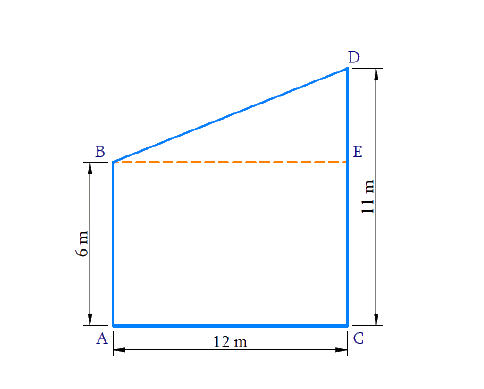### Solution

Reasoning:

In a right triangle, the square of the hypotenuse is equal to the sum of the squares of the other two sides.

Steps:

$$AB$$ is the height of one pole $$= 6\;\rm{}m$$

$$CD$$ is the height of another pole $$= 11\,\rm{}m$$

$$AC$$ is the distance between two poles at bottom $$= 12\rm{}\,m$$

$$BD$$ is the distance between the tops of the poles $$=\,?$$

Draw $$B E \| A C$$

Now consider,

In $$\Delta B E D$$

\begin{align}\angle B E D&=90^{\circ} \\ {BE}={AC}&=12 \mathrm{m}\\ {DE}&={CD}-\mathrm{CE} \\ {DE}&=11-6=5 \mathrm{cm}\\\end{align}

Now,

\begin{align} B D^{2} &=B E^{2}+D E^{2}\\ & [ \text {Pythagoras } \text{ theorem} ] \\\\ &=12^{2}+5^{2} \\ &=144+25 \\ B D^{2} &=169 \\ B D &=13 \mathrm{m} \end{align}

The distance between the tops of poles $$=13\rm{}\,m$$

## Chapter 6 Ex.6.5 Question 13

$$D$$ and $$E$$ are points on the sides $$CA$$ and $$CB$$ respectively of a triangle $$ABC$$ right angled at $$C$$. Prove that  \begin{align} A E^{2}+B D^{2}=A B^{2}+D E^{2}\end{align}.

Diagram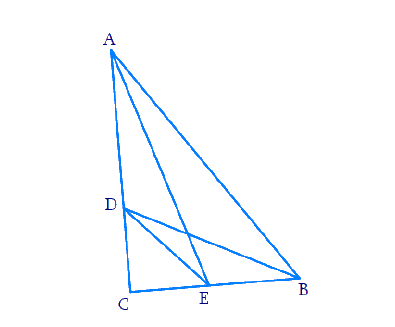### Solution

Reasoning:

In a right triangle, the square of the hypotenuse is equal to the sum of the squares of the other two sides.

Steps:

In $$\Delta ABC,\,\,\angle ABC={{90}^{\circ}}$$

$$D$$, $$E$$ are points on $$AC$$ and $$BC$$

Join $$AE$$, $$DE$$ and $$BD$$

In $$\Delta ACE$$ ,

\begin{align} AE{}^{2} & =A{{C}^{2}}+C{{E}^{2}} \;\;\dots (1) \\ & [ \text { Pythagoras } \text{ theorem } ] \end{align}

In $$\Delta DCB$$

\begin{align} BD{}^{2}=C{{D}^{2}}+B{{C}^{2}} \;\;\dots (2) \end{align}

Adding $$(1)$$ and $$(2)$$

\begin{align} & AE{}^{2}+B{{D}^{2}} \\ & \;\;= \! A{C}^{2}\!+ \! CE{}^{2} \! +\! C{D}^{2} \!\!+ \! B{{C}^{2}} \\ & \;\;= \! A{C}^{2} \! + \! BC{}^{2} \! + \! E{{C}^{2}} \! + \! C{{D}^{2}} \\ & \;\;=A{{B}^{2}}+DE{}^{2} \\ \end{align}

\begin{align} & \text{In}\,\,\Delta ABC,\,\,\,\,\, \angle C={{90}^{\circ}} \\ & \Rightarrow A{{C}^{2}}+BC{}^{2}=A{{B}^{2}} \\\\ & \qquad \qquad \text{and} \\\\ & \text{In}\,\,\Delta CDE,\,\,\angle DCE={{90}^{\circ}} \\ & \Rightarrow C{{D}^{2}}+C{{E}^{2}}=D{{E}^{2}}\\ &\Rightarrow A{{E}^{2}}+BD{}^{2}=A{{B}^{2}}+D{{E}^{2}}\ \end{align}

## Chapter 6 Ex.6.5 Question 14

The perpendicular from $$A$$ on side $$BC$$ of a $$\Delta ABC$$ intersects $$BC$$ at $$D$$ such that $$DB$$ $$=$$ $$3CD$$(see Fig. below). Prove that $$2A{{B}^{2}}=2A{{C}^{2}}+B{{C}^{2}}$$ .

Diagram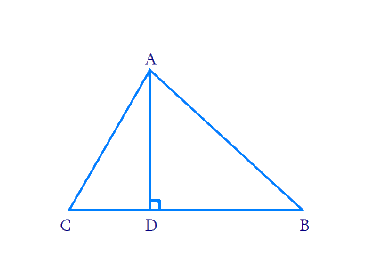### Solution

Reasoning:

In a right triangle, the square of the hypotenuse is equal to the sum of the squares of the other two sides.

Steps:

In $$\Delta ABC$$

$$AD\bot BC$$ and $$\,BD=3CD$$

\begin{align}BD + CD &= BC\\3CD + CD &= BC\\4CD &= BC\\CD &= \frac{1}{4}BC \ldots \left( 1 \right)\\\\&{\rm{and\qquad }}\\\\BD &= \frac{3}{4}BC \ldots \left( 2 \right)\end{align}

In $$\Delta ADC$$,

\begin{align}A{C^2} &= AD{}^2 + C{D^2}\\ & \quad \left[ {\angle ADC = {{90}^0}} \right]\\A{D^2} & = A{C^2} - C{D^2} \ldots .\left( 3 \right)\end{align}

In $$\Delta ADB$$,

\begin{align}A{B^2} &= AD{}^2 + B{D^2} \\ & \quad \left[ {\angle ADB = {{90}^0}} \right]\\A{B^2} &= A{C^2} - C{D^2} + B{D^2} \\ & \quad\left[ {{\rm{from (3)}}} \right]\\A{B^2}\,\, &= \!\! A{C^2} \! +\! {\left( \! {\frac{3}{4}BC} \! \right)^2} -\! {\left(\! {\frac{1}{4}BC}\! \right)^2} \\ & \quad\left[ {{\rm{from (1) and (2)}}}\right]\\ \\ A{B^2} &= A{C^2} + \frac{{9B{C^2} - B{C^2}}}{{16}}\\A{B^2} &= A{C^2} + \frac{{8B{C^2}}}{{16}}\\A{B^2} &= A{C^2} + \frac{1}{2}B{C^2}\\2A{B^2} &= 2A{C^2} + B{C^2}\end{align}

## Chapter 6 Ex.6.5 Question 15

In an equilateral triangle $$ABC$$, $$D$$ is a point on side $$BC$$ such that $$BD$$ $$=$$\begin{align}\frac{1}{3}BC\end{align}

Prove that $$9A{{D}^{2}}=7AB{}^{2}$$ .

Diagram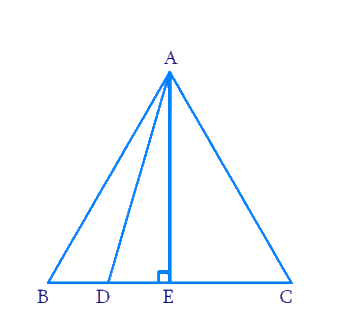### Solution

Reasoning:

In a right triangle, the square of the hypotenuse is equal to the sum of the squares of the other two sides.

Steps:

\begin{align} \text{In}\,\,\Delta A B C ; & \,\, A B=B C=C A \\ & \text{and}\,\,BD=\frac{1}{3}BC \\ & \text{Draw} \,AE\bot BC \\ & BE=CE=\frac{1}{2}BC \end{align}

[ $$\because$$ In an equilateral triangle perpendicular drawn from vertex to opposite side bisects the side]

Now In $$\Delta ADE$$

\begin{align} A{{D}^{2}} & =AE{}^{2}+D{{E}^{2}} \\ & [ \text { Pythagoras } \text{ theorem } ] \end{align}

\begin{align}=(\frac{\sqrt{3}}{2}BC)+{{(BE-BD)}^{2}}\end{align}

[ $$\because$$ $$AE$$ is the height of an equilateral triangle which is equal to \begin{align}\frac{\sqrt{3}}{2}\end{align} side]

\begin{align}A{D^2} &= \frac{3}{4}B{C^2} + {\left[ {\frac{{BC}}{2} - \frac{{BC}}{3}} \right]^2}\\A{D^2} &= \frac{3}{4}B{C^2} + {\left( {\frac{{BC}}{6}} \right)^2}\\ A{D^2} &= \frac{3}{4}B{C^2} + \frac{{B{C^2}}}{{36}}\\A{D^2} &= \frac{{27B{C^2} + B{C^2}}}{{36}}\\36A{D^2} &= 28B{C^2}\\9A{D^2} &= 7B{C^2}\\9A{D^2} &= 7A{B^2} \\& \left[ {AB \!= \!BC\! = \!CA} \right]\end{align}

## Chapter 6 Ex.6.5 Question 16

In an equilateral triangle, prove that three times the square of one side is equal to four times the square of one of its altitudes.

Diagram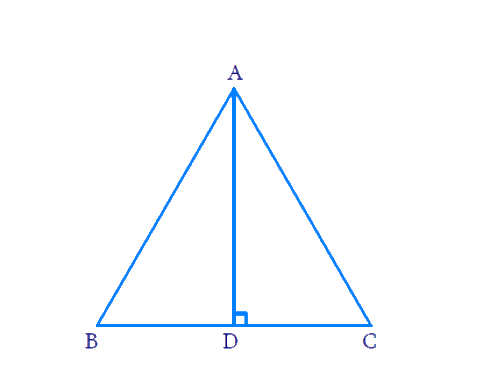We have to prove $$3B{{C}^{2}}=4A{{D}^{2}}$$

### Solution

Reasoning :

In a right triangle, the square of the hypotenuse is equal to the sum of the squares of the other two sides.

Steps:

In $$\Delta ABC$$

$$AB = BC = CA$$

\begin{align} & AD\perp BC \\ \Rightarrow & \;BD=CD=\frac{BC}{2} \end{align}

Now In $$\Delta ADC$$

\begin{align}& AC^2=AD^2+CD^2 \\ & BC^2=AD^2+\left(\frac{BC}{2}\right)^2 \\ & \left[AC=BC \;\; \operatorname{and} \;\;CD=\frac{BC}{2}\right] \\& {B C^{2}=A D^{2}+\frac{B C^{2}}{4}} \\& {B C^{2}-\frac{B C^{2}}{4}=A D^{2}} \\ &{\frac{3 B C^{2}}{4}=A D^{2}} \\ &{3 B C^{2}=4 A D^{2}}\end{align}

## Chapter 6 Ex.6.5 Question 17

Tick the correct answer and justify : In $$\Delta ABC$$, $$AB =$$ $$6\sqrt{3}\,\text{cm}$$ , $$AC =$$ $$12 \;\rm{}cm$$ and $$BC =$$ $$6 \;\rm{}cm$$. The angle $$B$$ is

(A) $$120^\circ$$

(B) $$60^\circ$$

(C) $$90^\circ$$

(D) $$45^\circ$$

### Solution

Reasoning:

In a right triangle, the square of the hypotenuse is equal to the sum of the squares of the other two sides.

Steps: ( c)

In $$\Delta ABC$$

\begin{align}AB&=6 \sqrt{3} \mathrm{cm} ;\\ AC&=12 \mathrm{cm} ; \\ BC & =6 \mathrm{cm} \\ \\ A B^{2}&=108 \mathrm{cm}^{2} ; \\ AC^{2} & =144 \mathrm{cm}^{2} ; \\ BC^{2} & =36 \mathrm{cm}^{2} \\\\ A B^{2}+B C^{2}&=(108+36) \mathrm{cm}^{2} \\ \quad&=144 \mathrm{cm}^{2} \\ \Rightarrow A C^{2}&=A B^{2}+B C^{2}\end{align}

Pythagoras theorem is satisfied

$\Rightarrow \angle ABC={{90}^{\circ}}$

Download SOLVED Practice Questions of NCERT Solutions For Class 10 Maths Chapter 6 Exercise 6.5 for FREE
Ncert Class 10 Exercise 6.5
Ncert Solutions For Class 10 Maths Chapter 6 Exercise 6.5
Instant doubt clearing with Cuemath Advanced Math Program